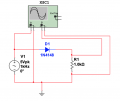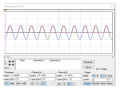Half wave rectifier

Pickles

Joined Mar 13, 2015
29
Good evening,

I am trying to complete some questions and I am struggling to understand what they are asking for. I have answered the other questions already but I'm not sure about these two.

The questions are:

Observe the traces of the input and output signals and comment on your results.

What is the average value of the input and output signal.
(Is this one asking for the average value of a sine wave?)

Below are the two images with it.Many thanks

MrChips

Joined Oct 2, 2009
25,037
The question asks for the average value of
1) the input signal
2) the output signal

How does one compute the average value?

You take the sum of N samples and divide by N.
For periodic signals, make sure that N samples fall within integer values of the period of the signal.
It is ok if the integer value = 1, i.e. sum over one period.

Do you know calculus?

•Zeeus

dl324

Joined Mar 30, 2015
13,838
Observe the traces of the input and output signals and comment on your results.
Since this is probably homework, what can you say about the signals?

What is the average value of the input and output signal.
(Is this one asking for the average value of a sine wave?)
It would seem to me that the question is also referring to the output waveform.

Delta Prime

Joined Nov 15, 2019
1,213
How does one compute the average value?
You're kidding me right!
Calculus is not needed. It's straightforward guys. We should consult short bus cuz everyone needs to be taken to school.

•Zeeus

djsfantasi

Joined Apr 11, 2010
8,075
You're kidding me right!
Calculus is not needed. It's straightforward guys. We should consult short bus cuz everyone needs to be taken to school.
There are straightforward methods, that approximate calculus. But it’s calculus that needs to be done. Have you been to school?

•Delta Prime and Zeeus

MrChips

Joined Oct 2, 2009
25,037
You're kidding me right!
Calculus is not needed. It's straightforward guys. We should consult short bus cuz everyone needs to be taken to school.
I am curious to see how you would do this without a knowledge of calculus.

•Delta Prime and djsfantasi

Delta Prime

Joined Nov 15, 2019
1,213
To do so would be giving the answer and I am on double secret probation take this thread out of homework.

SamR

Joined Mar 19, 2019
3,837
#1 Draw the signals for the supply V
#2 Draw the signal for the output V
#3 How do you calculate the average V for each signal???
It's pretty basic AC sine wave calculations that can be easily done without calculus... Although it was derived from calculus...

MisterBill2

Joined Jan 23, 2018
10,551
This looks like homework. The blue trace is the supply V voltage, the red trace is the waveform of the output V.
The formula for calculating the average value of a sine wave is tedious to derive.

djsfantasi

Joined Apr 11, 2010
8,075
This looks like homework. The blue trace is the supply V voltage, the red trace is the waveform of the output V.
The formula for calculating the average value of a sine wave is tedious to derive.
But to derive it, you need to know calculus, right?

MisterBill2

Joined Jan 23, 2018
10,551
But to derive it, you need to know calculus, right?
It was derived in my calculus class back in 1971, I recall. The solution for the relationship between the peak, RMS, and average voltages of a sine wave is just a simple numerical ratio multiplier. That number is easy to look up and use.

SamR

Joined Mar 19, 2019
3,837
And what happens to Vp after it crosses the diode?

•bertus

MisterBill2

Joined Jan 23, 2018
10,551
Ok thanks everyone. To work out the average value of the input and output signal would you use this equation...

View attachment 200318

Many thanks
That should be Vpp, the peak to peak voltage.

SamR

Joined Mar 19, 2019
3,837

Pickles

Joined Mar 13, 2015
29
And what happens to Vp after it crosses the diode?
Sorry I am really struggling with this. The notes we have been given don't explain anything.

Does any one know of an online circuit making website that I can build this circuit and see what is happening in it?

Thanks

Pickles

Joined Mar 13, 2015
29
The question asks for the average value of
1) the input signal
2) the output signal

How does one compute the average value?

You take the sum of N samples and divide by N.
For periodic signals, make sure that N samples fall within integer values of the period of the signal.
It is ok if the integer value = 1, i.e. sum over one period.

Do you know calculus?
I don't understand were you get any values from to work out the average.

Yes I can do Calculus.